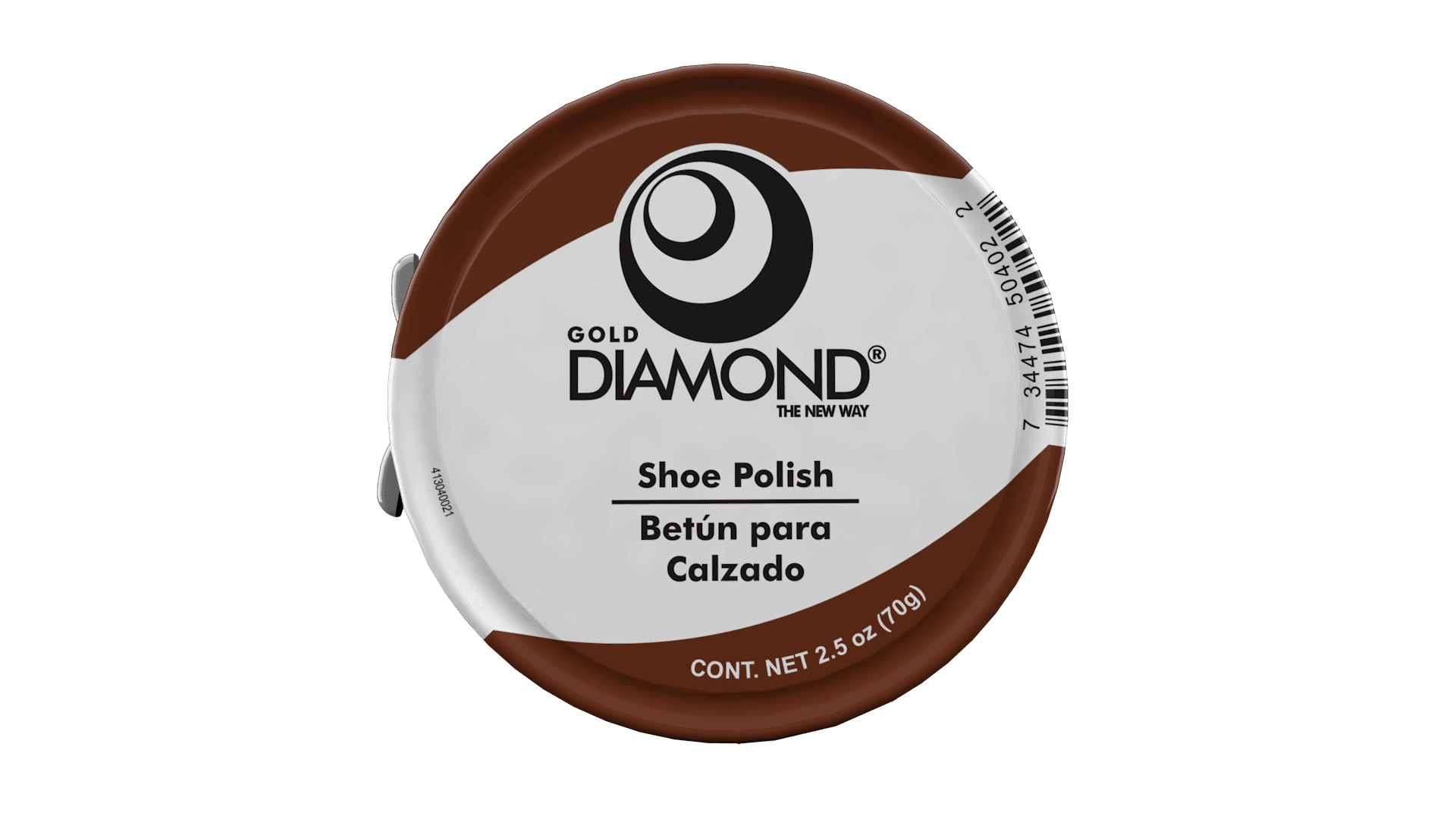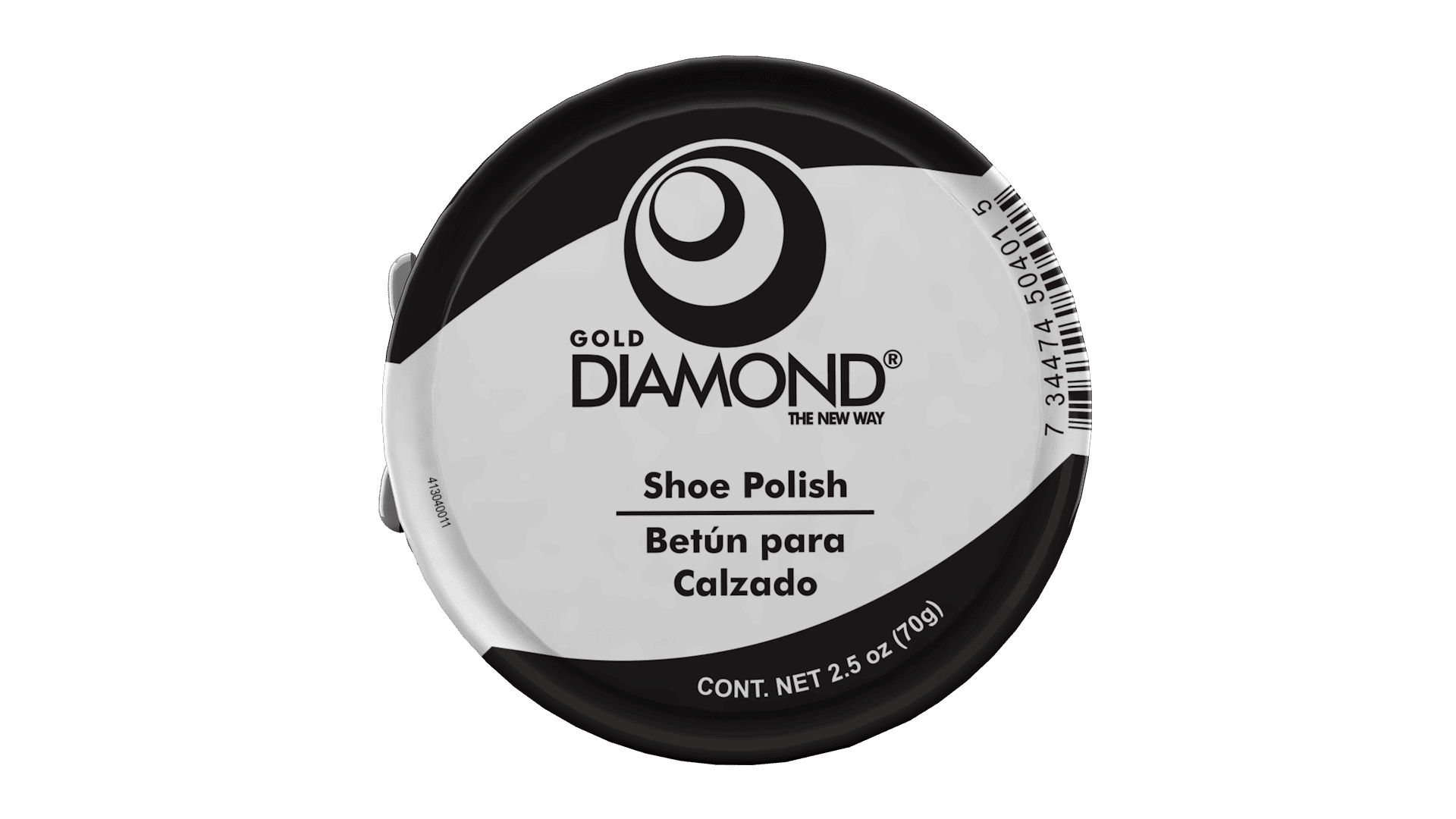# Polish Paste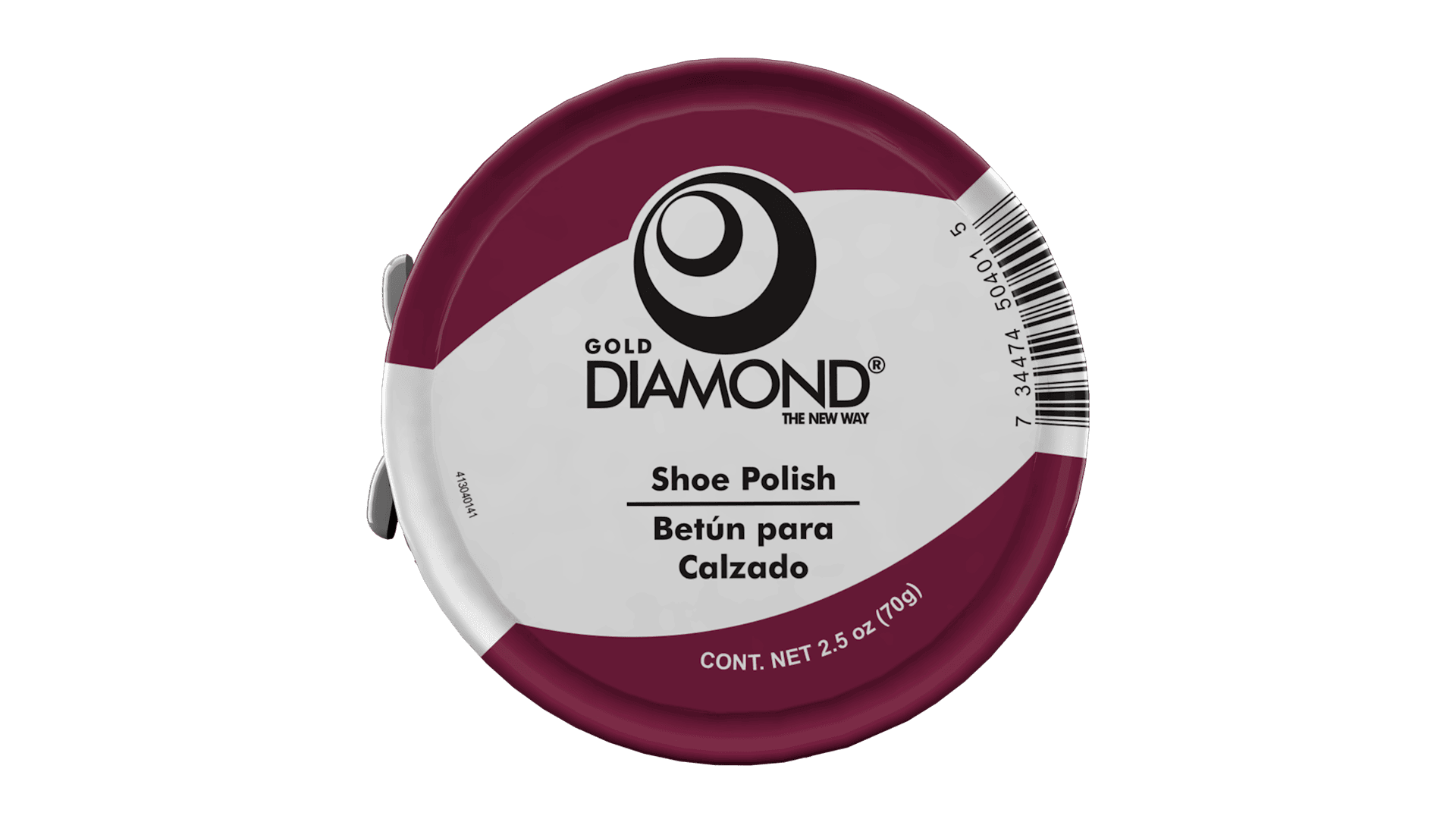## Polish Paste Wine

[cl_column width="1/2"][cl_clients 0="0="0="0="0="0="0="0="0="0="0="0="0="0="0="0="0="0="0="0="0="0="0="0="0="0="0="0="0="0="0="0="0="0="0="0="0="0="0="0="0="0="images="__array__"https://nterobon.sirv.com/Spins/Test%201/Test%201.spin",5186,5185__array__end__""""""""""""""""""""""""""""""""""""""""""" carousel="1" carousel_view_items="1" autoplay_timeout="3500" css_style="{'margin-top':'-15px'}_-_json" carousel_nav="1"] [cl_column width="1/2"] Gold Diamond Polish Paste Perfect combination of fine natural waxes, which provides shine and protection while also renewing footwear with each application. The shoe polish Gold Diamond provides lasting protection against [...]## Polish Paste M Brow

[cl_column width="1/2"][cl_clients 0="0="0="0="0="0="0="0="0="0="0="0="0="0="0="0="0="0="0="0="0="0="0="0="0="0="0="0="0="0="0="0="0="0="0="0="0="0="0="0="0="0="0="0="images="__array__"https://nterobon.sirv.com/Spins/Test%201/Test%201.spin",5186,5185__array__end__""""""""""""""""""""""""""""""""""""""""""""" carousel="1" carousel_view_items="1" autoplay_timeout="3500" css_style="{'margin-top':'-15px'}_-_json" carousel_nav="1"] [cl_column width="1/2"] Gold Diamond Polish Paste Perfect combination of fine natural waxes, which provides shine and protection while also renewing footwear with each application. The shoe polish Gold Diamond provides lasting protection against [...]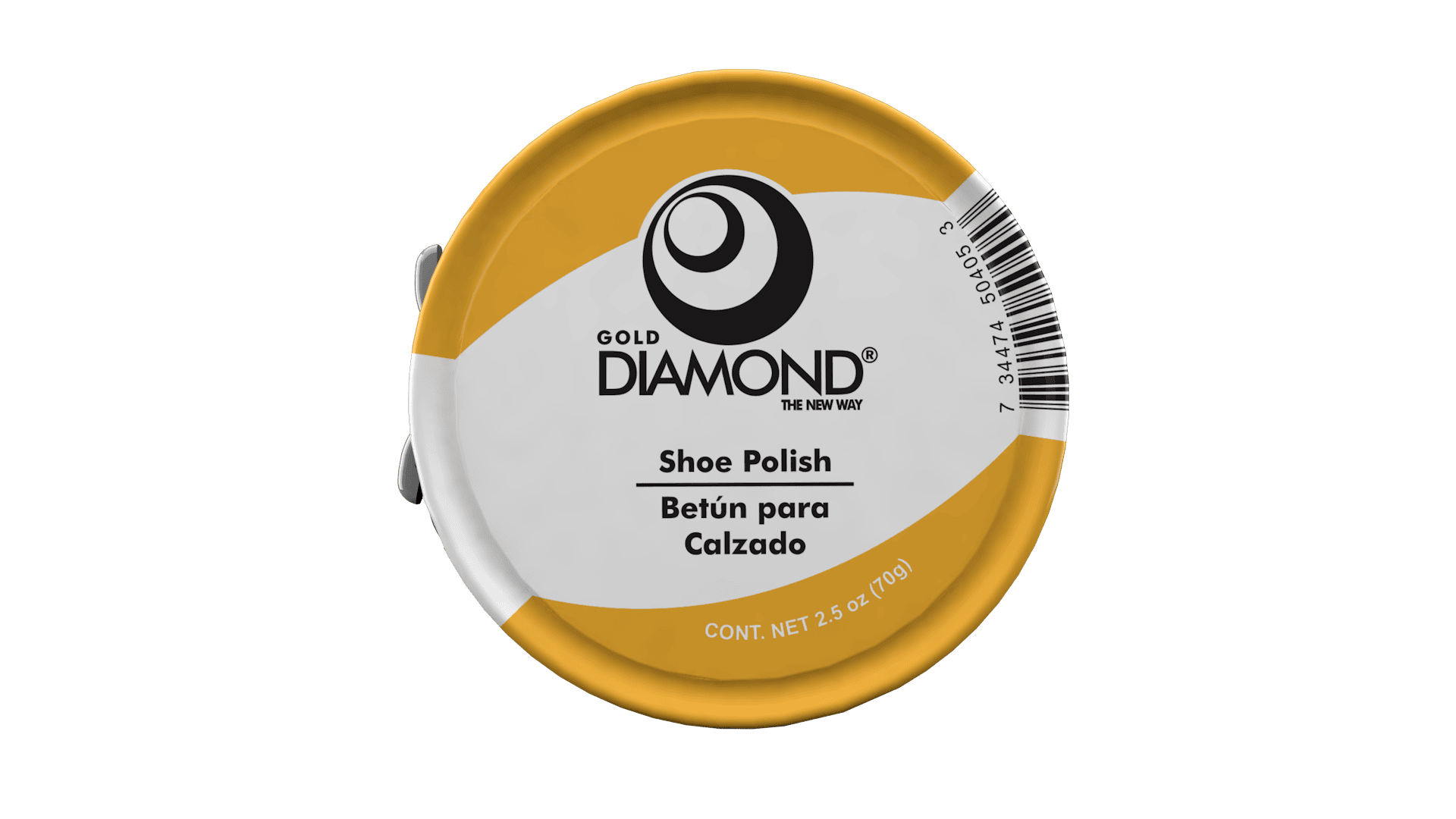## Polish Paste Yellow

[cl_column width="1/2"][cl_clients 0="0="0="0="0="0="0="0="0="0="0="0="0="0="0="0="0="0="0="0="0="0="0="0="0="0="0="0="0="0="0="0="0="0="0="0="0="0="0="0="0="0="0="0="0="images="__array__"https://nterobon.sirv.com/Spins/Test%201/Test%201.spin",5186,5185__array__end__"""""""""""""""""""""""""""""""""""""""""""""" carousel="1" carousel_view_items="1" autoplay_timeout="3500" css_style="{'margin-top':'-15px'}_-_json" carousel_nav="1"] [cl_column width="1/2"] Gold Diamond Polish Paste Perfect combination of fine natural waxes, which provides shine and protection while also renewing footwear with each application. The shoe polish Gold Diamond provides lasting protection against [...]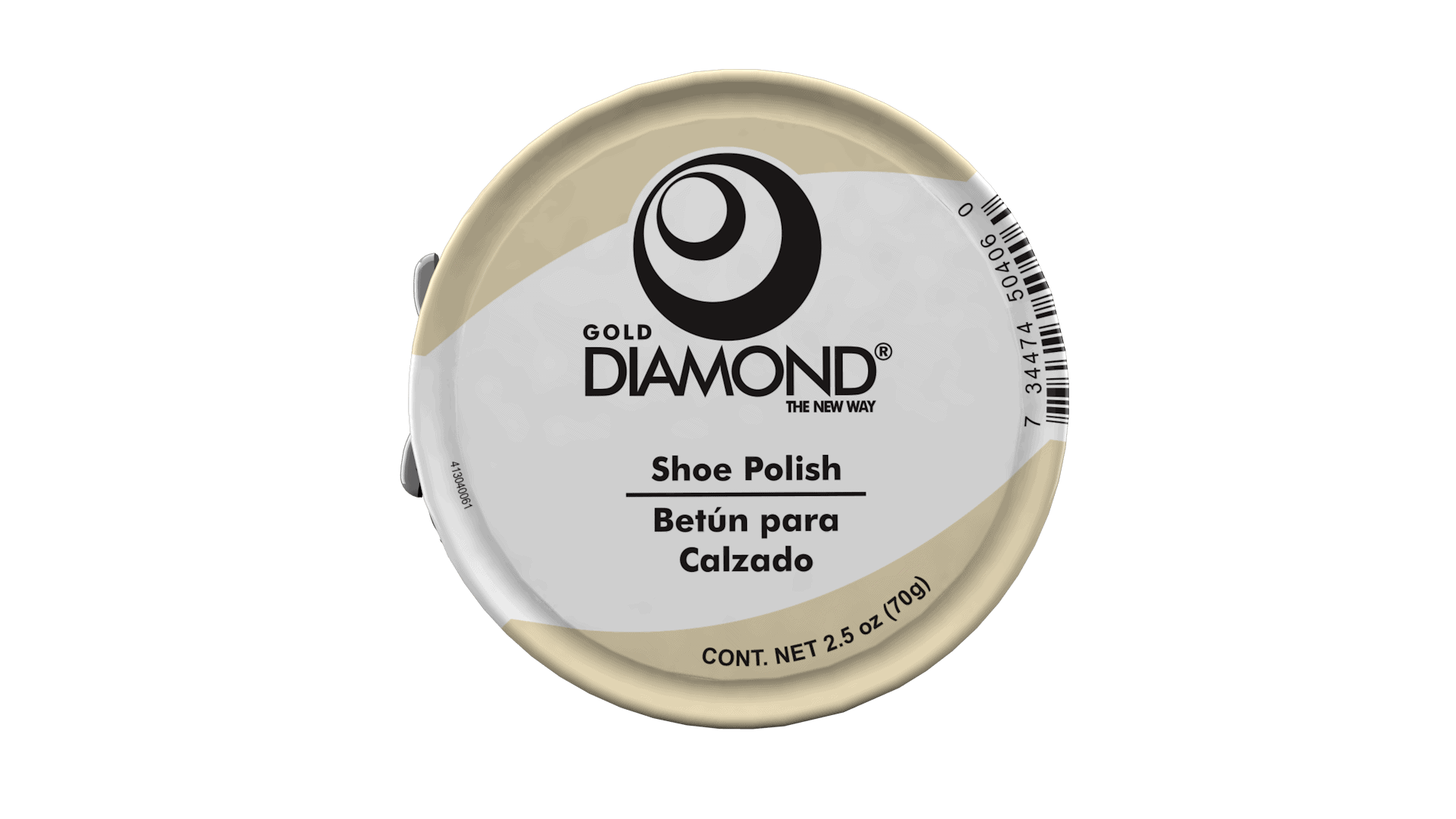## Polish Paste Neutral

[cl_column width="1/2"][cl_clients 0="0="0="0="0="0="0="0="0="0="0="0="0="0="0="0="0="0="0="0="0="0="0="0="0="0="0="0="0="0="0="0="0="0="0="0="0="0="0="0="0="0="0="0="0="images="__array__"https://nterobon.sirv.com/Spins/Test%201/Test%201.spin",5186,5185__array__end__"""""""""""""""""""""""""""""""""""""""""""""" carousel="1" carousel_view_items="1" autoplay_timeout="3500" css_style="{'margin-top':'-15px'}_-_json" carousel_nav="1"] [cl_column width="1/2"] Gold Diamond Polish Paste Perfect combination of fine natural waxes, which provides shine and protection while also renewing footwear with each application. The shoe polish Gold Diamond provides lasting protection against [...]